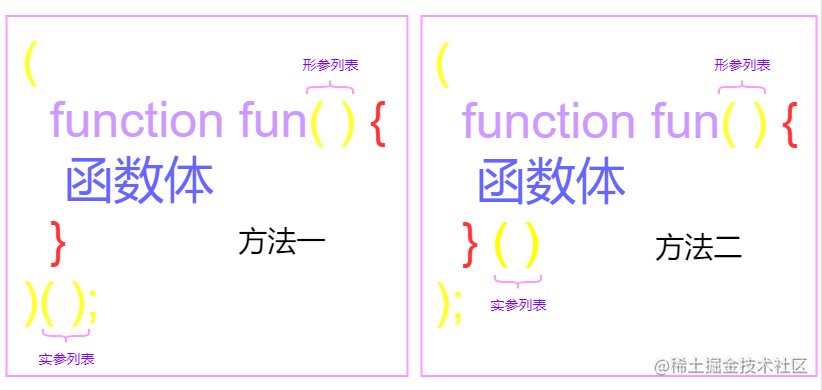JS高级之必会的5个高阶函数递归

1. 子问题跟原始问题一样，且更为简单
2. 必须有个出口

1. 通过使用函数名实现
2. 通过使用arguments.callee属性实现。

var v = 1 // 出口条件
function fun() {
console.log('第' + v + '次调用函数')
v++
if (v <= 3) {
fun()
}
}
fun()

回调函数

// 定义一个函数，这个函数有两个函数类型的参数，然后分别执行那两个函数，并返回它们的和。
function sum(a, b) {
// 目标函数
return a() + b()
}

function one() {
// 回调函数
return 1
}

function two() {
// 回调函数
return 2
}

console.log(sum(one, two)) // 3

匿名回调函数

// 定义一个函数，这个函数有两个函数类型的参数，然后分别执行那两个函数，并返回它们的和。
function sum(a, b) {
// 目标函数
return a() + b()
}

console.log(
sum(
function () {
// 匿名回调函数
return 1
},
function () {
//  匿名回调函数
return 2
},
),
) // 3

带参数的回调函数

function multiplyByTwo(list, callback) {
list.forEach(function (v, i) {
callback(v * 2, i)
})
}
var list = [1, 2, 3]
multiplyByTwo(list, function (v, i) {
list[i] = v
})
console.log(list) // [ 2, 4, 6 ]

回调函数的优缺点

• 匿名回调函数节省了全局命名空间
• 将私有的数据内容开放给指定位置使用
• 保证封装性——虽然可以使用私有数据，但是并不知道来源
• 有助于提升性能

自调函数

;(function () {
console.log('自调函数')
})();+(function (v) {
// 形参
var w = 100 // 局部变量
console.log('自调函数' + v)
})(1) // 实参

!(function (v) {
var w = 100 // 局部变量
console.log('自调函数' + v)
})(2)

~(function (v) {
var w = 100 // 局部变量
console.log('自调函数' + v)
})(3)

作为值的函数

function outer() {
var v = 100
// 在函数的函数体中定义另一个函数 -> 内部(私有)函数
return function () {
// 使用匿名函数
return v * 2
}
}
var result = outer()
console.log(result) // [Function]

• 有助于我们确保全局名字空间的纯净性（这意味着命名冲突的机会很小）。
• 确保私有性 — 这使我们可以选择只将一些必要的函数暴露给“外部世界”，而保留属于自己的函数，使它们不为该应用程序的其他部分所用。

闭包

1. 外部函数中定义了内部函数。
2. 外部函数是具有返回值，且返回值为内部函数。
3. 内部函数还引用了外部函数的变量。

1. 作用域没有那么直观。
2. 因为变量不会被垃圾回收所以有一定的内存占用问题。

1. 可以使用同级的作用域。
2. 读取其他元素的内部变量。
3. 延长作用域。
4. 避免污染全局变量

• 在预编译阶段，如果发现内部函数使用了外部函数的变量，它就会在内存中 创建一个闭包对象并保存相对应的值，如果已经存在闭包，则只需要增加对应属性值即可。
• 在执行完成后，函数执行上下文会被校徽，函数对闭包对象的引用也会被销毁，但其内部函数还持有该闭包的引用，所以内部函数还可以继续使用外部函数的变量

// 1. 通过返回的内部函数来操作函数中的局部变量
function fun () {
var v = 100; // 局部变量
// 通过返回一个对象的方式访问局部变量v 来完成闭包
return {
set: function (x) {
v = x;
},
get: function () {
return v
}
}
}
var result = fun();
result.set(200)
console.log(result.get()); // 200

// 2. 定义一个局部变量，计算该函数一共调用几次
var generate_count = function () {
var container = 0;
return function () {
container++
console.log(`这是第\${container}次调用`);
}
}

var result = generate_count();
result(); // 这是第1次调用
result(); // 这是第2次调用
result(); // 这是第3次调用

// 3.修改 Math.pow() 函数，让求一个数的平方或者立方时，不需要每次传递第二个参数

/*
Math.pow(4, 2) // 求4的平方
Math.pow(4, 3) // 求4的立方
*/

// 写一个函数生成器
function makePower (power) {
return (number) => {
return Math.pow(number, power)
}
}
// 平方
let power2 = makePower(2)
// 立方
let power3 = makePower(3)
// 求4的平方
console.log(power2(4)) // 16
// 求4的立方
console.log(power3(4)) // 62